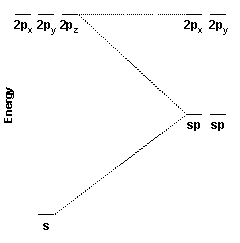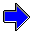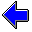Java Security Settings:
This web page employs Java, which requires specific security settings for correct operation.
If the applets on this page do not run correctly, consult the Virtual Chemistry Experiments FAQ
or the Physlet Physics web site for establishing the correct security settings.

Visualization of Atomic Orbitals

Hybrid Orbitals

In Valence Bond Theory, a chemical bond between two atoms is the result of direct overlap of two atomic orbitals (one on each atom). For a sigma bond, the overlap is along the line directly between the two nuclei. Good orbital overlap requires that the atomic orbitals on each atom (those orbitals overlapping to form the bond) be oriented directly toward the other atom.

The basic s, px, py, and pz orbitals are unsatisfactory for two reasons. First, these orbitals are not directed in a particular direction; instead, they tend to spread out in all directions (or at least multiple directions). Second, to the extent that the orbitals have an orientation (the px along the x axis, for example), the geometry is often inconsistent with the molecular geometry. For example, in methane (CH4) the carbon is at the center of the molecule and the hydrogens lie on the points of a tetrahedron. Each H-C-H bond angle is 109.5o. The carbon 2s orbital is spherical and as such extends toward all four hydrogen atoms. The carbon 2px, 2py, and 2pz extend along the x, y, and z axes and as such form 90o angles with each other. This geometry does not provide for effective overlap with the hydrogen atoms.

The solution to this problem is to create a new set of atomic orbitals. Recall that the Schrödinger equation for the hydrogen atom is a linear differential equation. Any linear combination of solutions to this equation is also a solution. Thus for n = 2, there are an infinite number of solutions, not just the 2s, 2px, 2py, and 2pz wave functions. The carbon atom, in the example given above, will choose the set of wave functions that minimizes its energy. For an isolated carbon, the 2s, 2px, 2py, and 2pz wave functions are optimal, but in the presence of other atoms, where chemical bonding can occur, an alternate set of orbitals may be preferred.

Valence Bond Theory manages this situation by creating hybrid orbitals that are linear combinations of the s, px, py, and pz orbitals in the valence shell. (The d orbitals may also be included, if necessary.) The hybrid orbitals have energies intermediate between those of the basic orbitals used to construct them. Basic atomic orbitals not employed in constructing the hybrid orbitals are unaffected by the hybridization process.

sp Hybrid Orbital

 Consider the carbon dioxide molecule. The O-C-O bond angle in CO2 is 180o; the molecule is perfectly linear. Valence Bond Theory requires two orbitals on the carbon atom that can be used to form sigma bonds with the two oxygens. One hybrid orbital provides direct overlap with an orbital on one oxygen atom; the other hydrid orbital provides direct overlap with an orbital on the other oxygen atom. These two hybrid orbitals, the sp hybrid orbitals, are constructed from the carbon 2s and 2pz orbitals. Because two atomic orbitals were used to create the hybrid orbitals, two hybrid orbitals are formed. One sp hybrid orbital is oriented along the positive z axis; the other is oriented in the opposite direction. The energy diagram for the hybridization process is shown at the right. Notice that the px and py orbitals are unaffected while the s and pz orbitals are been converted into two new degenerate orbitals of intermediate energy.Carbon 2s Orbital Carbon sp Hybrid Orbital Carbon 2pz Orbital

sp2 Hybrid Orbital

 Consider the carbonate ion. The O-C-O bond angle in CO32- is 120o; the molecule is perfectly planar. Valence Bond Theory requires three orbitals on the carbon atom that can be used to form sigma bonds with the three oxygens. For each oxygen atom, there is one sp2 hybrid orbital on the oxygen that provides direct overlap with an orbital on the oxygen atom. These three hybrid orbitals, the sp2 hybrid orbitals, are constructed from the carbon 2s, 2pz, and 2py orbitals. Because three atomic orbitals were used to create the hybrid orbitals, three hybrid orbitals are formed. One sp2 hybrid orbital is oriented along the positive z axis, one is oriented 120o to the left, and the other is oriented 120o to the right.Carbon 2s Orbital Carbon sp2 Hybrid Orbital Carbon 2pz Orbital

Other Hybrid Orbital

Other sets of hybrid orbitals can be constructed to accomodate other geometries. The table below provides a list of common geometries and sets of hybrid orbitals having this geometry.

Geometry Hybrid Orbitals Number of Orbitals Atomic Orbitals used to form Hybrid Orbitals
linear sp 2 s, pz
trigonal planar sp2 3 s, py, pz
tetrahedral sp3 4 s, px, py, pz
trigonal bipyramidal dsp3 5 s, px, py, pz, dz2
octahedral d2sp3 6 s, px, py, pz, dz2, dx2-y2
square planar dsp2 4 s, px, py, dx2-y2

The electron density plots below compare the sp, sp2, and sp3 orbitals. Notice that these orbitals are all very similar, in that the majority of the orbital is oriented in a particular direction. A p orbital is distributed equally in two opposite directions (e.g., half in the positive z direction and half in the negative z direction). As the amount of p character in the hybrid orbital increases, the hybrid orbital also develops a more symmetric distribution.

 sp Hybrid Orbital sp2 Hybrid Orbital sp3 Hybrid Orbital

Hybrid orbitals based on d orbitals are only possible for elements in the third or higher rows, and the structures of these orbitals are more complicated.

The dsp3 hybrid orbitals, which taken together have trigonal bipyramidal geometry, are somewhat unusual in that not all orbitals are identical. The axial orbitals, which are oriented along the z axis, are different from the equitorial orbitals, which lie in the xy plane.

 dsp3 Equitorial Hybrid Orbital dsp3 Axial Hybrid Orbital d2sp3 Hybrid OrbitalGeometry of Hybrid OrbitalsAtomic Orbitals Home PageVirtual Chemistry Experiments Home Page

hybrid.html version 2.1